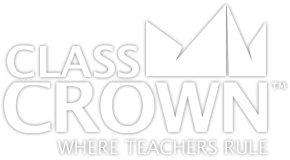# Decimals Worksheets

## Decimals Worksheets: Pack 1

Skills included in this pack:
• Naming place value to the thousandths place
• Decimals expressed as pictures
• Decimals expressed in words
• Rounding decimals to the hundredths place
• Identifying decimals on a number line
• Comparing decimals
• Decimals expressed as fractions
• Comparing decimals with fractions
• Subtracting decimals
• Adding and subtracting decimals word problems
• Multiplying decimals by powers of 10
• Multiplying decimals by one-digit whole numbers
• Multiplying decimals by two-digit whole numbers
• Representing multiplication of decimals visually
• Multiplying decimals
• Dividing decimals by powers of 10
• Dividing decimals by whole numbers
• Dividing with decimals in the divisor
• Division: adding a decimal and zeros to the dividend
+ See More
Free Sample Pages Available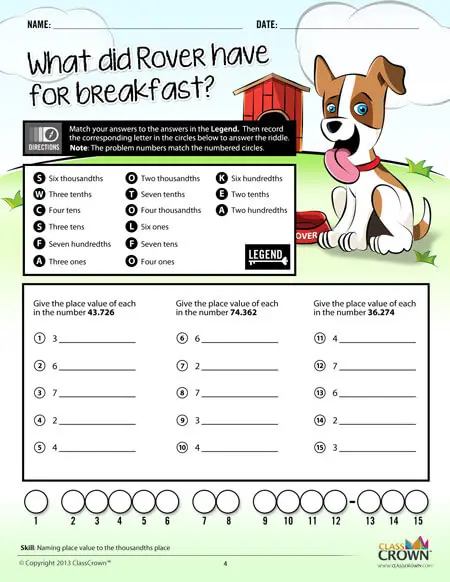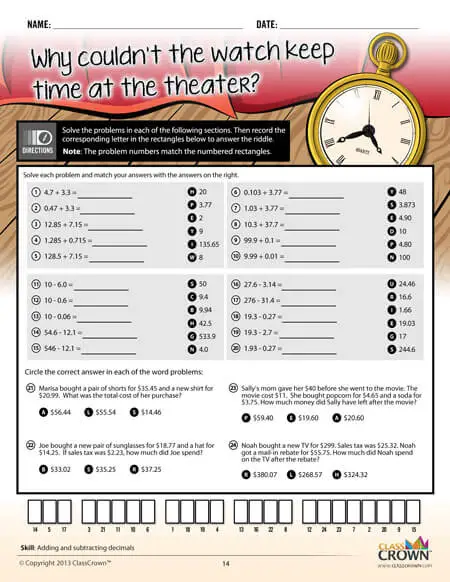## 4th Grade Math Worksheets: Pack 1

Skills included in this pack:
• Interpreting multiplication
• Multiplying or dividing to solve word problems involving multiplication comparison
• Interpreting remainders in division word problems
• Solving word problems with four operations
• Solving two-step problems
• inding factors for whole numbers between 1 and 100
• Identifying factors and multiples
• Identifying prime numbers
• Prime factorization
• Determining if a number is a multiple
• Continuing patterns
• Creating a pattern with a given rule
• Multiplication with multiples of 10
• Examining whole number digit place value
• Using place value to solve multiplication & division problems
• Converting between expanded form and numeric form
• Converting between numeric number and word form
• Comparing numbers using <, >, and =
• Rounding to a given place
• Adding up to four digit numbers
• Subtracting up to four digit numbers
• Multiplying using arrays with factors of 10
• Multiplying using arrays
• Multiplying up to four digits by one digit
• Dividing by one digit with remainders
• Dividing with partial quotients
• Estimating quotients
• Dividing three-digit numbers by one-digit numbers with remainder
• Dividing using visual representation
• Division word problems with and without remainders
+ See More
Free Sample Pages Available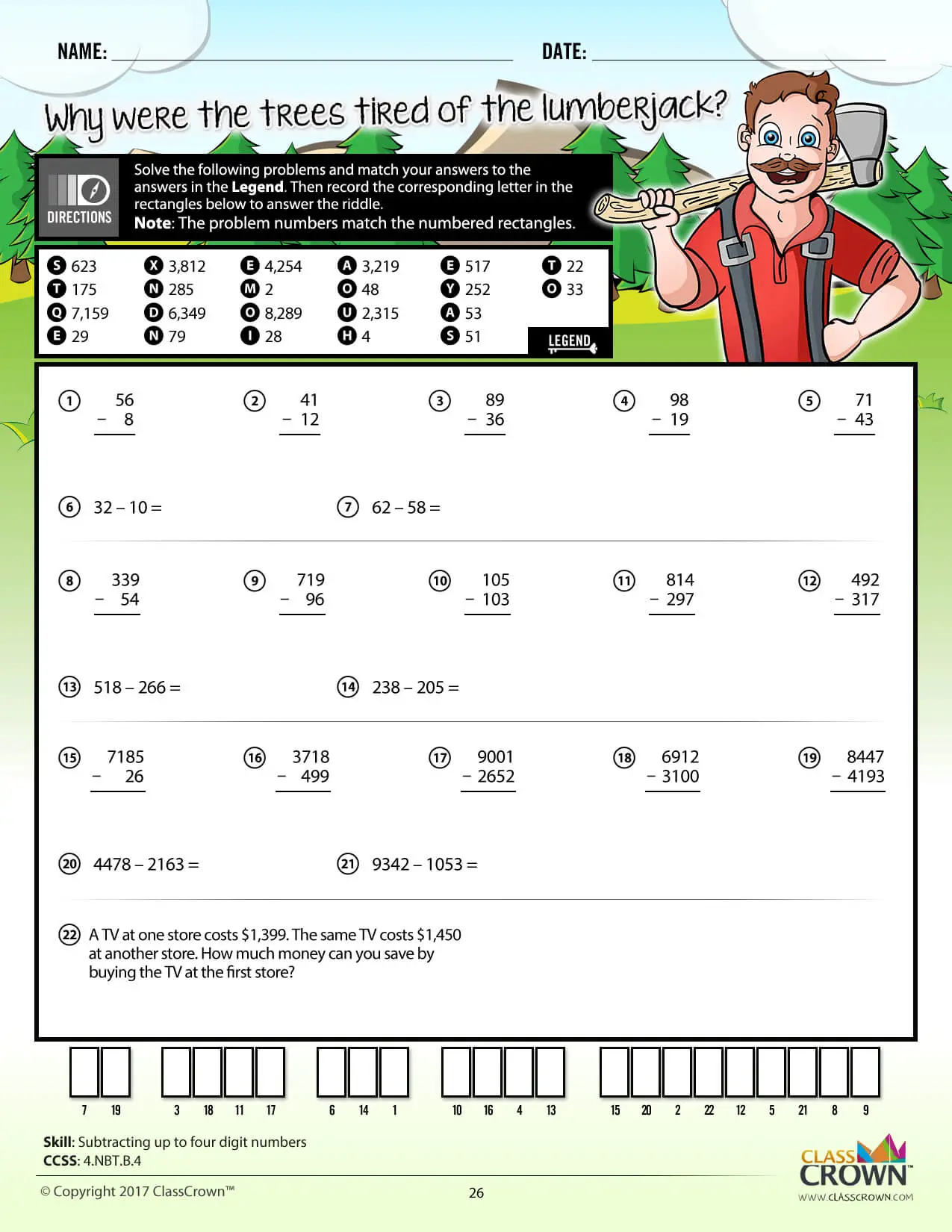Self Checking

Our math worksheets introduce a puzzle aspect to math, giving students immediate feedback as to whether or not they are solving problems correctly. If the answer to the riddle isn't spelled correctly, the student knows which problems he's made an error on.Fun Puzzle AspectImmediate Feedback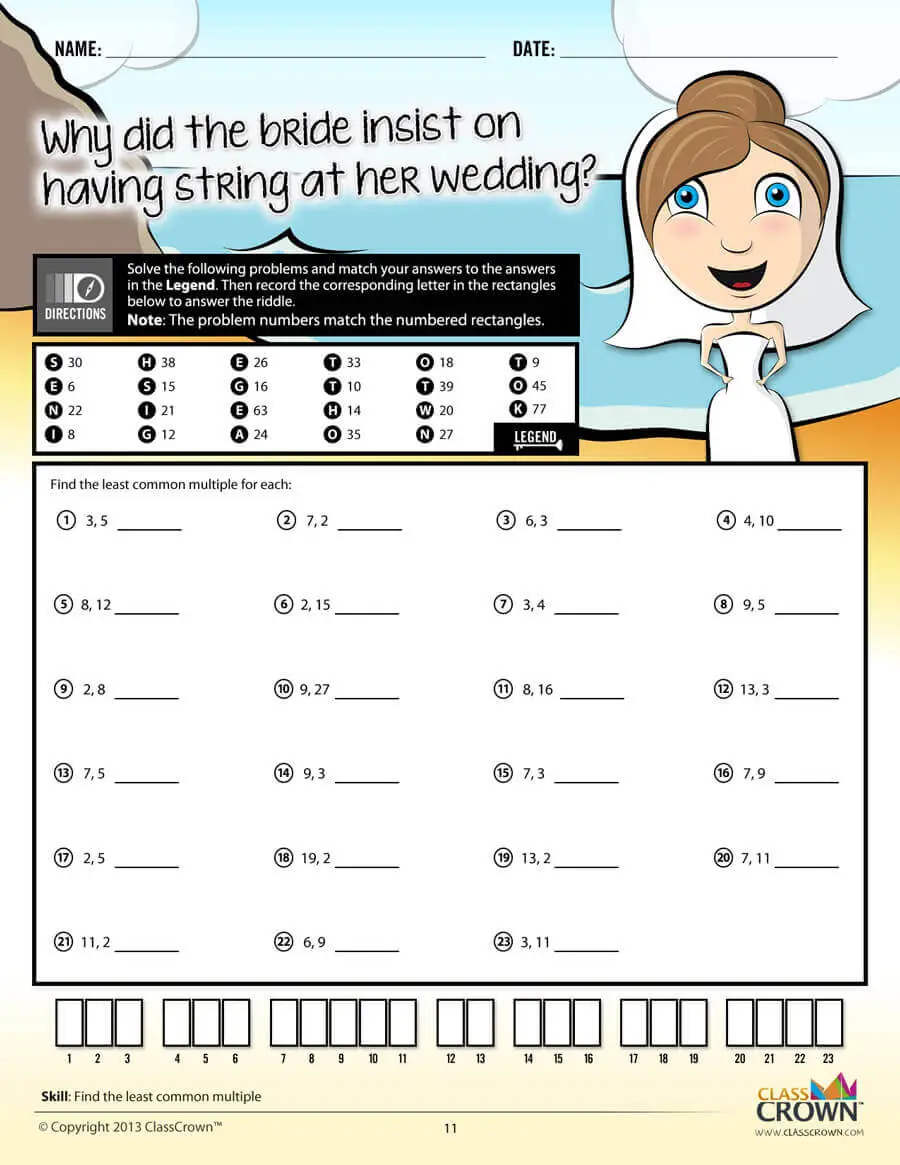Problem Solving Motivation

Each math worksheet contains a riddle that the student solves by completeing all the problems on the worksheet. This keeps kids motivated to complete each problem so that they can find the answer to the riddle.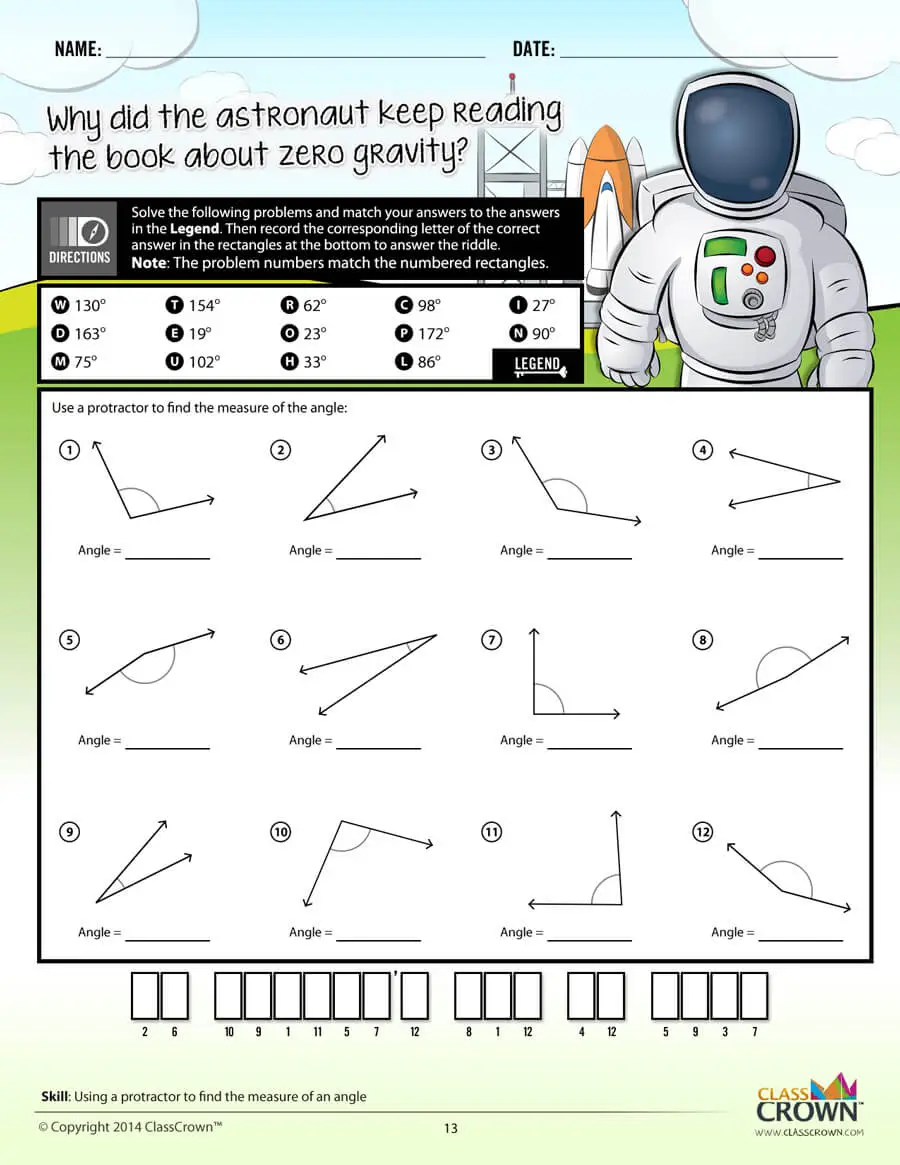Common Core Aligned

All our math worksheet packs are designed with Common Core in mind. That way you don’t have to worry about whether your math ciriculum is aligned or not when you incorpoate ClassCrown Riddle-Me-Worksheets in your lesson plans.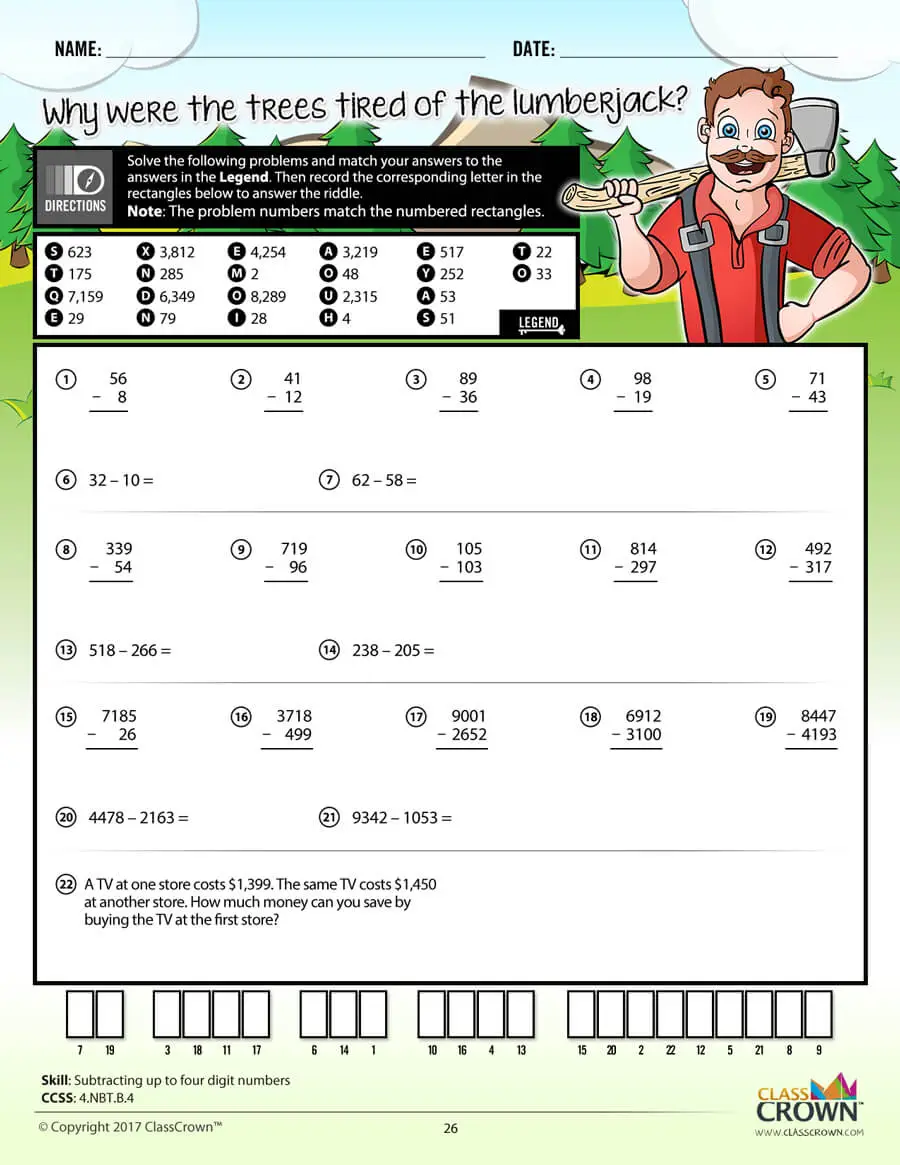High Quality Design

Each page of our math worksheets has been produced in high resolution at 144 dpi and designed in full, vibrant color for maximum quality. They look stunning whether you are printing in color or black and white.High Resolution
(144 dpi)Stunning Color & Clarity